# More on quantum entanglement and irreducibility

A few posts ago, I talked about how quantum mechanics entails the existence of irreducible states – states of particles that in principle cannot be described as the product of their individual components. The classic example of such an entangled state is the two qubit state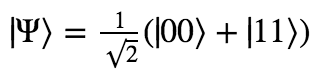This state describes a system which is in an equal-probability superposition of both particles being |0 and both particles being |1. As it turns out, this state cannot be expressed as the product of two single-qubit states.

A friend of mine asked me a question about this that was good enough to deserve its own post in response. Start by imagining that Alice and Bob each have a coin. They each put their quarter inside a small box with heads facing up. Now they close their respective boxes, and shake them up in the exact same way. This is important! (as well as unrealistic) We suppose that whatever happens to the coin in Alice’s box, also happens to the coin in Bob’s box.

Now we have two boxes, each of which contains a coin, and these coins are guaranteed to be facing the same way. We just don’t know what way they are facing.

Alice and Bob pick up their boxes, being very careful to not disturb the states of their respective coins, and travel to opposite ends of the galaxy. The Milky Way is 100,000 light years across, so any communication between the two now would take a minimum of 100,000 years. But if Alice now opens her box, she instantly knows the state of Bob’s coin!

So while Alice and Bob cannot send messages about the state of their boxes any faster than 100,000 years, they can instantly receive information about each others’ boxes by just observing their own! Is this a contradiction?

No, of course not. While Alice does learn something about Bob’s box, this is not because of any message passed between the two. It is the result of the fact that in the past the configurations of their coins were carefully designed to be identical. So what seemed on its face to be special and interesting turns out to be no paradox at all.

Finally, we get to the question my friend asked. How is this any different from the case of entangled particles in quantum mechanics??

Both systems would be found to be in the states |00 and |11⟩ with equal probability (where |0⟩ is heads and |1⟩ is tails). And both have the property that learning the state of one instantly tells you the state of the other. Indeed, the coins-in-boxes system also has the property of irreducibility that we talked about before! Try as we might, we cannot coherently treat the system of both coins as the product of two independent coins, as doing so will ignore the statistical dependence between the two coins.

(Which, by the way, is exactly the sort of statistical dependence that justifies timeless decision theory and makes it a necessary update to decision theory.)

I love this question. The premise of the question is that we can construct a classical system that behaves in just the same supposedly weird ways that quantum systems behave, and thus make sense of all this mystery. And answering it requires that we get to the root of why quantum mechanics is a fundamentally different description of reality than anything classical.

So! I’ll describe the two primary disanalogies between entangled particles and “entangled” coins.

# Epistemic Uncertainty vs Fundamental Indeterminacy

First disanalogy. With the coins, either they are both heads or they are both tails. There is an actual fact in the world about which of these two is true, and the probabilities we reference when we talk about the chance of HH or TT represent epistemic uncertainty. There is a true determinate state of the coins, and probability only arises as a way to deal with our imperfect knowledge.

On the other hand, according to the mainstream interpretation of quantum mechanics, the state of the two particles is fundamentally indeterminate. There isn’t a true fact out there waiting to be discovered about whether the state is |00⟩ or |11⟩. The actual state of the system is this unusual thing called a superposition of |00⟩ and |11⟩. When we observe it to be |00⟩, the state has now actually changed from the superposition to the determinate state.

We can phrase this in terms of counterfactuals: If when we look at the coins, we see that they are HH, then we know that they were HH all along. In particular, we know that if we had observed them a moment later or earlier, we would have gotten H with 100% certainty. Give that we actually observed HH, the probability that we would have observed HH is 100%.

But if we observe the state of the particles to be |00⟩, this does not mean that had we observed it a moment before, we would be guaranteed to get the same answer. Given that we actually observed |00⟩, the probability that we would have observed |00⟩ is still 50%.

(A project for some enterprising reader: see what the truths of these counterfactuals imply for an interpretation of quantum mechanics in terms of Pearl-style causal diagrams. Is it even possible to do?)

## Predictive differences

The second difference between the two cases is a straightforward experimental difference. Suppose that Alice and Bob identically prepare thousands of coins as we described before, and also identically prepare thousands of entangled particles. They ensure that the coins are treated exactly the same way, so that they are guaranteed to all be in the same state, and similarly for the entangled pairs.

If they now just observe all of their entangled pairs and coins, they will get similar results – roughly half of the coins will be HH and roughly half of the entangled pairs will be |00⟩. But there are other experiments they could run on the entangled pairs that would give different answers depending on whether we treat the particles as being in superposition or not. I described what these experiments could be in this earlier post – essentially they involve applying an operation that takes qubits in and out of superposition. The conclusion of this is that even if you tried to model the entangled pair as a simple probability distribution similar to the coins, you will get the wrong answer in some experiments.

So we have both a theoretical argument and a practical argument for the difference between these two cases. They key take-away is the following:

According to quantum mechanics an entangled pair is in a state that is fundamentally indeterminate. When we describe it with probabilities, we are not saying “This probabilistic description is an account of my imperfect knowledge of the state of the system”. We’re saying that nature herself is undecided on what we will observe when we look at the state. (Side note: there is actually a way to describe epistemic uncertainty in quantum mechanics. It is called the density matrix, and is distinct from the description of superpositions.)

In addition, the most fundamental and accurate probability description for the state of the two particles is one that cannot be described as the product of two independent particles. This is not the case with the coins! The most fundamental and accurate probability description for the state of the two coins is either 100% HH or 100% TT (whichever turns out to be the case). What this means is that in the quantum case, not only is the state indeterminate, but the two particles are fundamentally interdependent – entangled. There is no independent description of the individual components of the system, there is only the system as a whole.

# Communication through entanglement

Is it possible to use quantum entanglement to communicate faster than light?

Here’s a suggestion for how we might achieve just such a thing. Two people each possess one qubit of an entangled pair in the state ⟩:The owner of the second qubit then decides whether or not to apply some quantum gate U to their qubit. Immediately following this, the owner of the first qubit measures their qubit.

If the application of U to the second qubit changes the amplitude distribution over the first qubit, then the measurement can be used to communicate a message between the two people instantaneously! Why? Well, initially 0 and 1 are expected with equal probability. But if applying U makes these probabilities unequal, then the observation of a 0 or 1 carries evidence as to whether or not U was applied. (In an extreme case, applying U could make it guaranteed that the qubit would be observed as |0⟩, in which case observation of |1⟩ ensures that U was not applied.)

In this way, somebody could send information across by encoding it in a string of decisions about whether or not to apply U to a shared entangled pair.

It might seem a little strange that doing something to your qubit over here could affect the state of their qubit over there. But this is quantum mechanics, and quantum mechanics is very, very strange. Remember that the two qubits are entangled with one another. We are already guaranteed that what happens to one can affect the state of the other instantaneously – after all, if we measure the second qubit and find it in the state |0⟩, the first qubit’s state is instantaneously “collapsed” into the state |0⟩ as well. (This fact alone cannot be used to communicate messages, because before measuring the second qubit, its owner has no control over which of the two states it will end up in.)

So whether or not our scheme will work cannot be ruled out a priori. We must work out the math for ourselves to see if applying U to qubit 2 can successfully warp the probability distribution of qubit 1, thus sending information between the two instantaneously.

First, we’ll describe our single qubit gate U as a matrix.a, b, c, and d are complex numbers. Can they have any possible values?

No. U must preserve the normalization of states it acts on. In other words, for U to represent a physically possible transformation of a qubit, it cannot transform physically possible states into physically impossible states.

What precise constraints does this entail? It turns out that the following two suffice to ensure the normalization condition:Alright. Now, we have our general description of a single-qubit gate. Of course, the qubit that U is operating on is a part of an entangled pair. It is an irreducible component of a two-qubit system. So we can’t actually describe it as just a single qubit.

Instead, we need to describe the state of both qubits, which, I’ll remind you, looks like: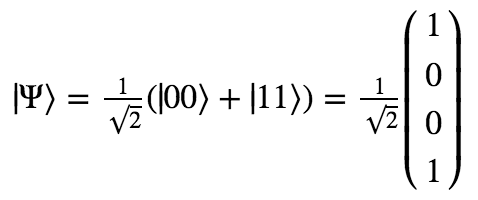A 2×2 matrix can’t operate on a vector with four components. What we need is a two-qubit quantum gate that corresponds to applying U to qubit 2 while leaving qubit 1 alone. “Leaving a qubit alone” is equivalent to applying the identity gate I to it, which just leaves the state unchanged.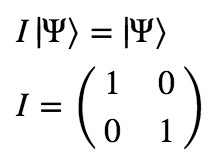So what we really want is the 4×4 matrix that corresponds to applying U to qubit 2 and I to qubit 1. It turns out that we can generate this matrix by simply taking the tensor product of U with I:Alright, now we’re ready to see what happens when we apply this gate to ⟩!This state sure looks different than the state we started with! But is it different enough to have carried some information between the qubits? Let’s now look at the probabilities for measuring qubit 1 in the states 0 and 1: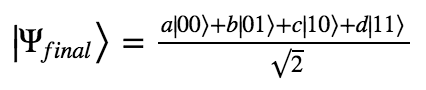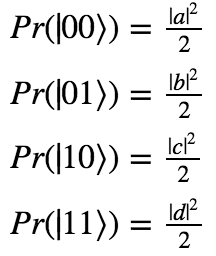Remember the constraints on the possible values of a, b, c, and d we started with?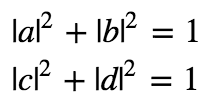Sadly, it looks like our method won’t work to produce faster-than-light communication. No matter what gate we apply to the second qubit, it has no effect on the observed probabilities of the first. And therefore, no information can be sent by applying U.

Of course, this does not rule out all possible ways to attempt to utilize entanglement to communicate faster-than-light. But it does provide a powerful demonstration of the way in which such attempts are defeated by the laws of quantum mechanics.

# Quantum mechanics, reductionism, and irreducibility

Take a look at the following two qubit state:Is it possible to describe this two-qubit system in terms of the states of the two individual particles that compose it? The principle of reductionism suggests to us that yes it should be possible; after all, of course we can always take any larger system and describe it perfectly fine in terms of its components.

But this turns out to not be the case! The above state is a perfectly allowable physical configuration of two particles, but there is no accurate description of the state of the individual particles composing the system!

Multi-particle systems cannot in general be reduced to their parts. This is one of the shocking features of quantum mechanics that is extremely easy to prove, but is rarely emphasized in proportion to its importance. We’ll prove it now.

Suppose we have a system composed of two qubits in states |Ψ1⟩ and |Ψ2⟩. In general, we may write:Now, as we’ve seen in previous posts, we can describe the state of the two qubits as a whole by simply smushing them together as follows:So the set of all two-qubit states that can be split in their component parts is the set of all states arising from all possible values of α1, α2, β1, and β2 such that all states are normalized. I.e.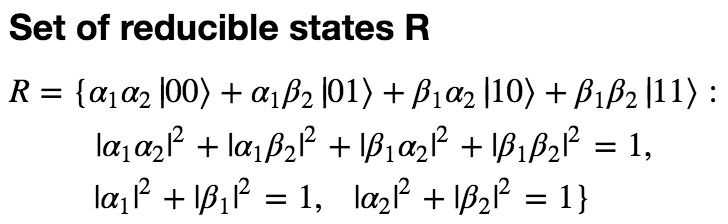However, there’s also a theorem that says that if any two states are physical possible, then all normalized linear combinations are physically possible as well. Because the states |00⟩, |01⟩, |10⟩, and |11⟩ are all physically possible, and because they form a basis for the set of two-qubit states, we can write out the set of all possible states:Now the philosophical question of whether or not there exist states that are irreducible can be formulated as a precise mathematical question: Does A = R?

And the answer is no! It turns out that A is much much larger than R.

The proof of this is very simple. R and A are both sets defined by a set of four complex numbers, and they share a constraint. But R also has two other constraints, independent of the shared constraint. That is, the two additional constraints cannot be derived from the first (try to derive it yourself! Or better, show that it cannot be derived). So the set of states that satisfy the conditions necessary to be in R must be smaller than the set of states that satisfy the conditions necessary to be in A. This is basically just the statement that when you take a set, and then impose a constraint on it, you get a smaller set.

An even simpler proof of the irreducibility of some states is to just give an example. Let’s return to our earlier example of a two-qubit state that cannot be decomposed into its parts:Suppose that ⟩ is reducible. Then for both |00⟩ and |11⟩ to have a nonzero amplitude, there must be a nonzero amplitude for the first qubit to be in the state |0⟩ and for the second to be in the state |1⟩. But then there can’t be zero amplitude for the state |01⟩. Q.E.D.!

More precisely:So here we have a two-qubit state that is fundamentally irreducible. There is literally no possible description of the individual qubits on their own. We can go through all possible states that each qubit might be in, and rule them out one.

Let’s pause for a minute to reflect on how totally insane this is. It is a definitive proof that according to quantum mechanics, reality cannot necessarily be described in terms of its smallest components. This is a serious challenge to the idea of reductionism, and I’m still trying to figure out how to adjust my worldview in response. While the notion of reductionism as “higher-level laws can be derived as approximations of the laws of physics” isn’t challenged by this, the notion that “the whole is always reducible to its parts” has to go.

In fact, I’ll show in the next section that if you try to make predictions about an system but analyze it in terms of its smallest components, you will not in general get the right answer.

# Predictive accuracy requires holism

So suppose that we have two qubits in the state we already introduced:You might think the following: “Look, the two qubits are either both in the state |0, or both in the state |1⟩. There’s a 50% chance of either one happening. Let’s suppose that we are only interested in the first qubit, and don’t care what happens with the second one. Can’t we just say that the first qubit is in a state with amplitudes 1/√2 in both states |0⟩ and |1⟩? After all, this will match the experimental results when we measure the qubit (50% of the time it is |0⟩ and 50% of the time it is |1⟩.”

Okay, but there are two big problems with this. First of all, while it’s true that each particle has a 50% chance of being observed in the state |0⟩, if you model these probabilities as independent of one another, then you will end up concluding that there is a 25% chance of the first particle being in the state |0⟩ and the second being in the state |1⟩. Whereas in fact, this will never happen!

You may reply that this is only a problem if you’re interested in making predictions about the state of the second qubit. If you are solely looking at your single qubit, you can still succeed at predicting what will happen when you measure it.

Well, fine. But the second, more important point is that even if you are able to accurately describe what happens when you measure your single qubit, you can always construct a different experiment you could perform that this same description will give the wrong answer for.

What this comes down to is the observation that quantum gates don’t operate the same way on 1/√2 (|00⟩ + |11⟩) as on 1/√2 (|0⟩ + |1⟩).

Suppose you take your qubit and pretend that the other one doesn’t exist. Then you apply a Hadamard gate to just your qubit and measure it. If you thought that the state was initially 1/√2 (|0⟩ + |1⟩), you will now think that your qubit is in the state |0⟩. You will predict with 100% confidence that if you measure it now, you will observe |0⟩.

But in fact when you measure it, you will find that 50% of the time it is |0⟩ and 50% of the time it is |1⟩! Where did you go wrong? You went wrong by trying to describe the particle as an individual entity.

Let’s prove this. First we’ll figure out what it looks like when we apply a Hadamard gate to only the first qubit, in the two-qubit representation: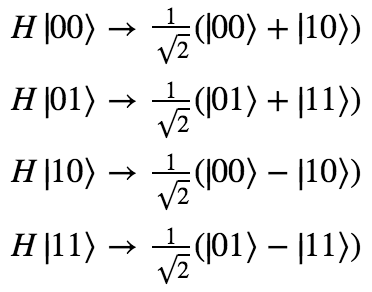So we have a ​25% chance of observing each of |00⟩|10⟩|01⟩, and|11⟩. Looking at just your own qubit, then, you have a 50% chance of observing |0⟩ and a 50% chance of observing |1⟩.

While your single-qubit description told you to predict a 100% chance of observing |0⟩, you actually would get a 50% chance of |0⟩ and a 50% chance of |1⟩.

Okay, but maybe the problem was that we were just using the wrong amplitude distribution for our single qubit. There are many choices we could have made for the amplitudes besides 1/√2 that would have kept the probabilities 50/50. Maybe one of these correctly simulates the behavior of the qubit in response to a quantum gate?

But no. It turns out that even though it is correct that there is a 50/50 chance of observing the qubit to be |0⟩ or |1⟩, there is no amplitude distribution matching this probability distribution that will correctly predict the results of all possible experiments.

Quick proof: We can describe a general two-qubit state with a 50/50 probability of being observed in |0⟩ and |1⟩ as follows: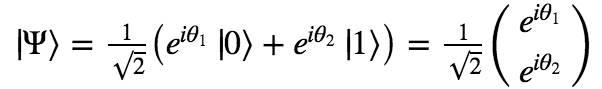For any ⟩, we can construct a specially designed quantum gate U that transforms ⟩ into |0⟩:Applying U to our single qubit, we now expect to observe |0⟩ with 100% probability. But now let’s look at what happens if we consider the state of the combined system. The operation of applying U to only the first qubit is represented by taking the tensor product of U with the identity matrix I: U ⊗ I.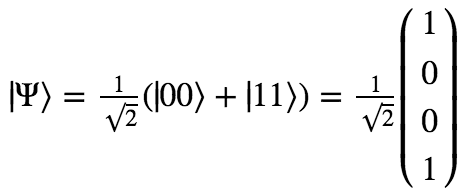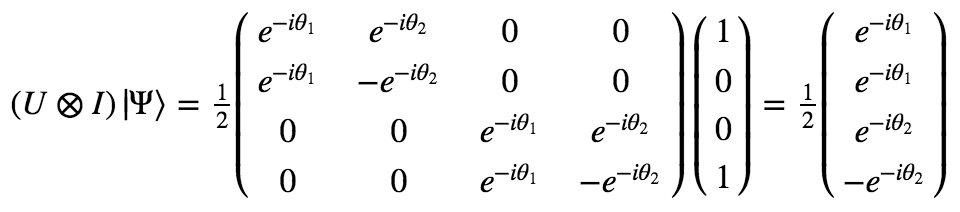What we see is that the two-qubit state ends up with a 25% chance of being observed as each of |00⟩, |01⟩, |10⟩, and |11⟩. This means that there is still a 50% chance of the first qubit being observed as |0⟩ and |1⟩.

This means that for every possible single qubit description of the first qubit, we can construct an experiment that will give different results than the model predicts. And the only model that always gives the right experimental predictions is a model that considers the two qubits as a single unit, irreducible and impossible to describe independently.

To recap: The lesson here is that for some quantum systems, if you describe them in terms of their parts instead of as a whole, you will necessarily make the wrong predictions about experimental results. And if you describe them as a whole, you will get the predictions spot on.

# So how many states are irreducible?

Said another way, how much larger is A (the set of all states) than R (the set of reducible states)? Well, they’re both infinite sets with the same cardinality (they each have the cardinality of the continuum, |ℝ|). So in this sense, they’re the same size of infinity. But we can think about this by considering the dimensionality of these various spaces.

Let’s take another look at the definitions of A and R:Each set is defined by four complex numbers, or 8 real numbers. If we ignored all constraints, then, our sets would be isomorphic to ℝ8.

Now, each share the same first constraint, which says that the overall state must be normalized. This constraint cuts one dimension off of the space of solutions, making it isomorphic to ℝ7.

That’s the only constraint for A, so we can say that A ~ ℝ7. But R involves two further constraints (the normalization conditions for each individual qubit). So we have three total constraints. However, it turns out that one of them can be derived from the others – two normalized qubits, when smushed together, always produce a normalized state. This gives us a net two constraints, meaning that the space of reducible states is isomorphic to ℝ6.

The space of irreducible states is what’s left when we subtract all elements of R from A. The dimensionality of this is just the same as the dimensionality of A. (A 3D volume minus a plane is still a 3D volume, a plane minus a curve is still two dimensional, a curve minus a point is still one dimensional, and so on.)

So both the space of total states and the space of irreducible states are 7-real-dimensional, while the space of reducible states is 6-real dimensional.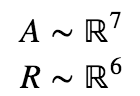You can visualize this as the space of all states being a volume, through which cuts a plane that composes all reducible states. The entire rest of the volume is the set of irreducible states. Clearly there are a lot more irreducible states than reducible states.

What about if we consider totally reducible three-qubit states? Now things are slightly different.

The set of all possible three qubit states (which we’ll denote A3) is a set of 8 complex numbers (16 real numbers) with one normalization constraint. So A3 ~ ℝ15.

The set of all totally reducible three qubit states (which we’ll denote R3) is a set of only six complex numbers. Why? Because we only need to specify two complex numbers for each of the three individual qubits that will be smushed together. So we start off with only 12 real numbers. Then we have three constraints, one for the normalization of each individual qubit. And the final normalization constraint (of the entire system) follows from the previous three constraints. In the end, we see that R3 ~ ℝ9.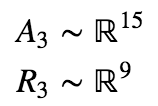Now the space of reducible states is six-dimensions less than the space of all states.

How does this scale for larger quantum systems? Let’s look in general at a system of N qubits.

AN is a set of 2N complex amplitudes (2N+1 real numbers), one for each N qubit state. There is just one normalization constraint. Thus we have a space with 2N+1 – 1 real dimensions.

On the other hand, RN is a set of only 2N complex amplitudes (4N real numbers), two for each of the N individual qubits. And there are N independent constraints ensuring that all states are normalized. So we have: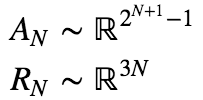The point of all of this is that as you consider larger and larger quantum systems, the dimensionality of the space of irreducible states grows exponentially, while the dimensionality of the space of reducible states only grows linearly. If we were to imagine randomly selecting a 20-qubit state from the space of all possibilities, we would be exponentially more likely to ending up with a space that cannot be described as a product of each of its parts.

What this means is that irreducibility is not a strange exotic phenomenon that we shouldn’t expect to see in the real world. Instead, we should expect that basically all systems we’re surrounded by are irreducible. And therefore, we should expect that the world as a whole is almost certainly not describable as the sum of individual parts.

# Building the diffusion operator

Part of the quantum computing series
Part 1: Quantum Computing in a Nutshell
Part 2: More on quantum gates
Part 3: Deutch-Josza Algorithm
Part 4: Grover’s algorithm
Part 5: Building the quantum oracle

Let’s more precisely define the Grover diffusion operator D we used for Grover’s algorithm, and see why it functions to flip amplitudes over the average amplitude.

First off, here’s a useful bit of shorthand we’ll use throughout the post. We define the uniform superposition over states as |s⟩:We previously wrote that flipping an amplitude ax over the average of all amplitudes ā involved the transformation ax → 2ā – ax. This can be understood by a simple geometric argument: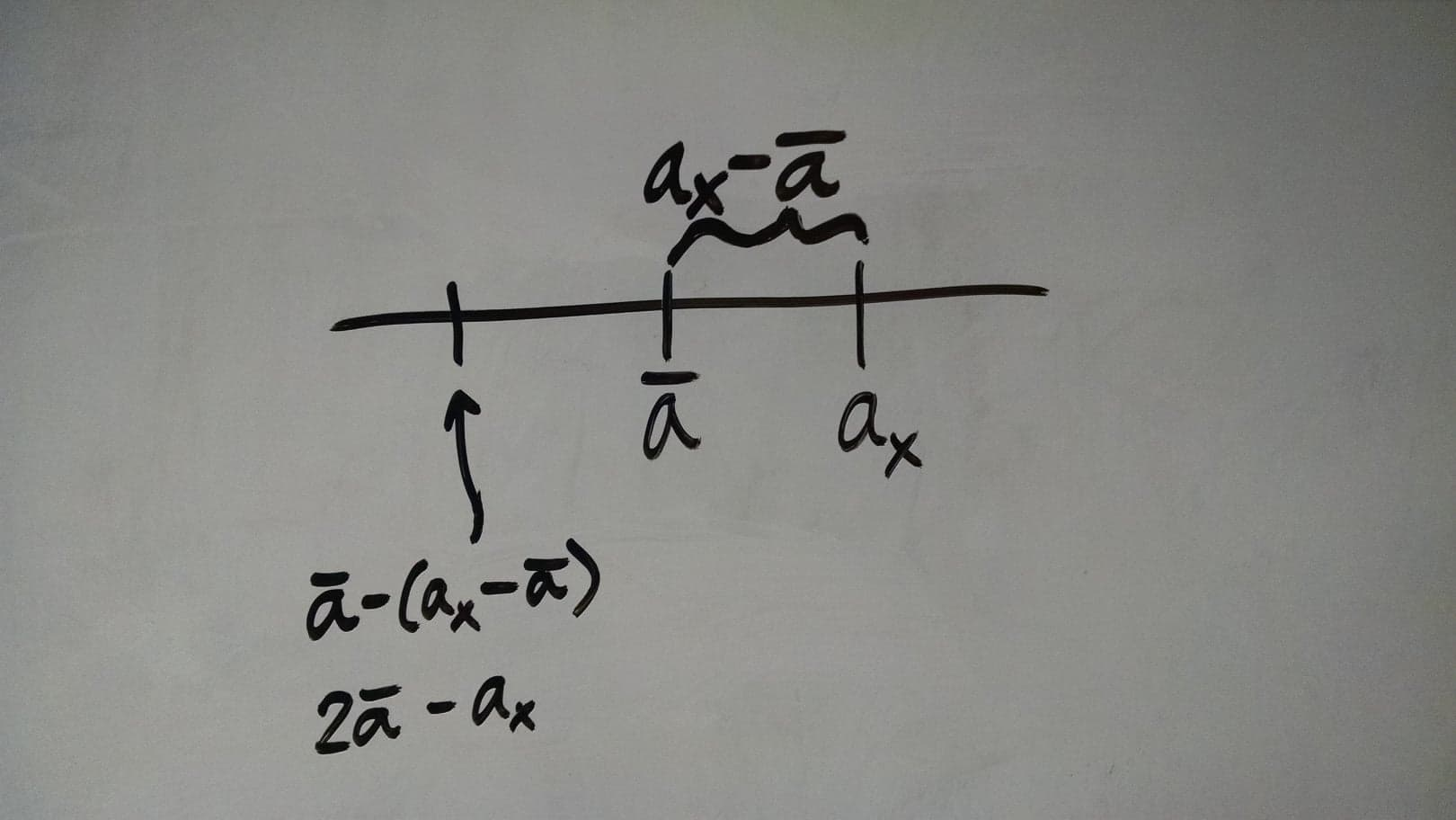Now, the primary challenge is to figure out how to build a quantum gate that returns the average amplitude of a state. In other words, we want to find an operator A such that acting on a state ⟩ gives:If we can find this operator, then we can just define D as follows: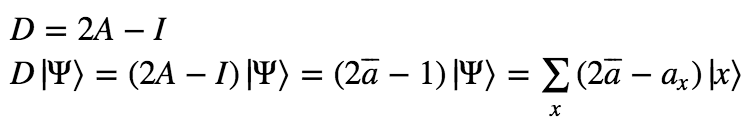It turns out that we can define A solely in terms of the uniform superposition.As a matrix, A would look like: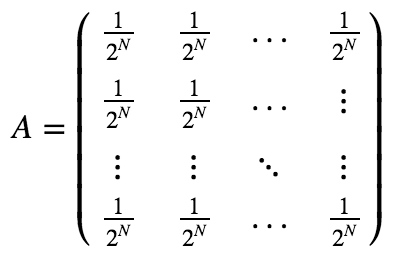Proof that this satisfies the definition: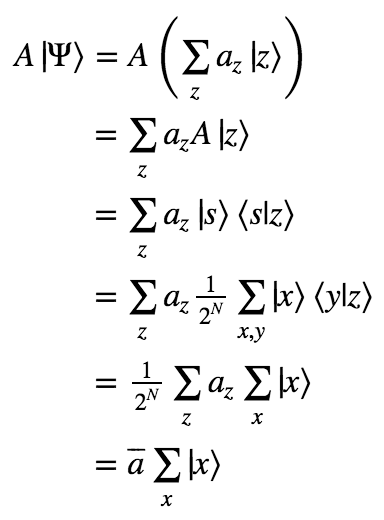Thus we have our full definition of D!As a matrix, D looks like:# Building the quantum oracle

Part 1: Quantum Computing in a Nutshell
Part 2: More on quantum gates
Part 3: Deutch-Josza Algorithm
Part 4: Grover’s algorithm

The quantum gate Uf was featured centrally in both of the previous algorithms I presented. Remember what it does to a qubit in state |x⟩, where x ∈ {0, 1}N: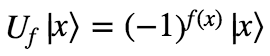I want to show here that this gate can be constructed from a simpler more intuitive version of a quantum oracle. This will also be good practice for getting a deeper intuition about how quantum gates work.

This will take three steps.

First we need to be able implement addition modulo 2 of two single qubits. This operation is defined as follows:An implementation of this operation as a quantum gate needs to return two qubits instead of just one. A simple choice might be: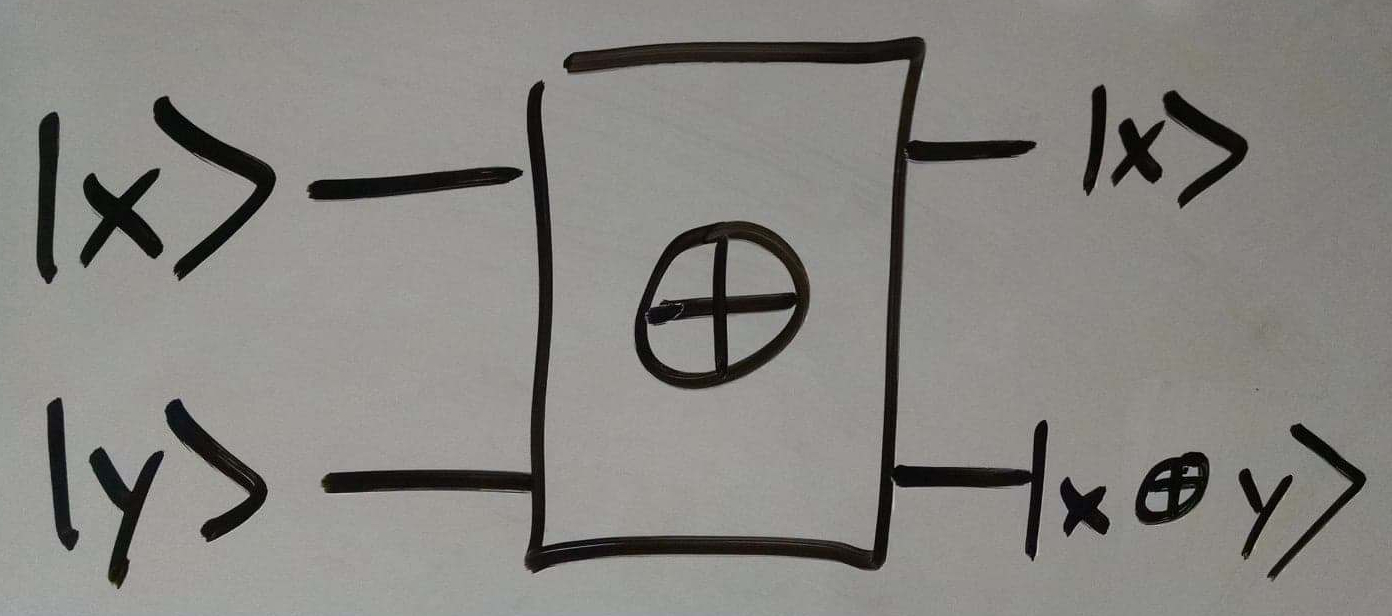## 2. Oracle

Next we’ll need a straight-forward implementation of the oracle for our function f as a quantum gate. Remember that f is a function from {0, 1}N → {0, 1}. Quantum gates must have the same number of inputs and outputs, and f takes in N bits and returns only a single bit, so we have to improvise a little. A simple implementation is the following: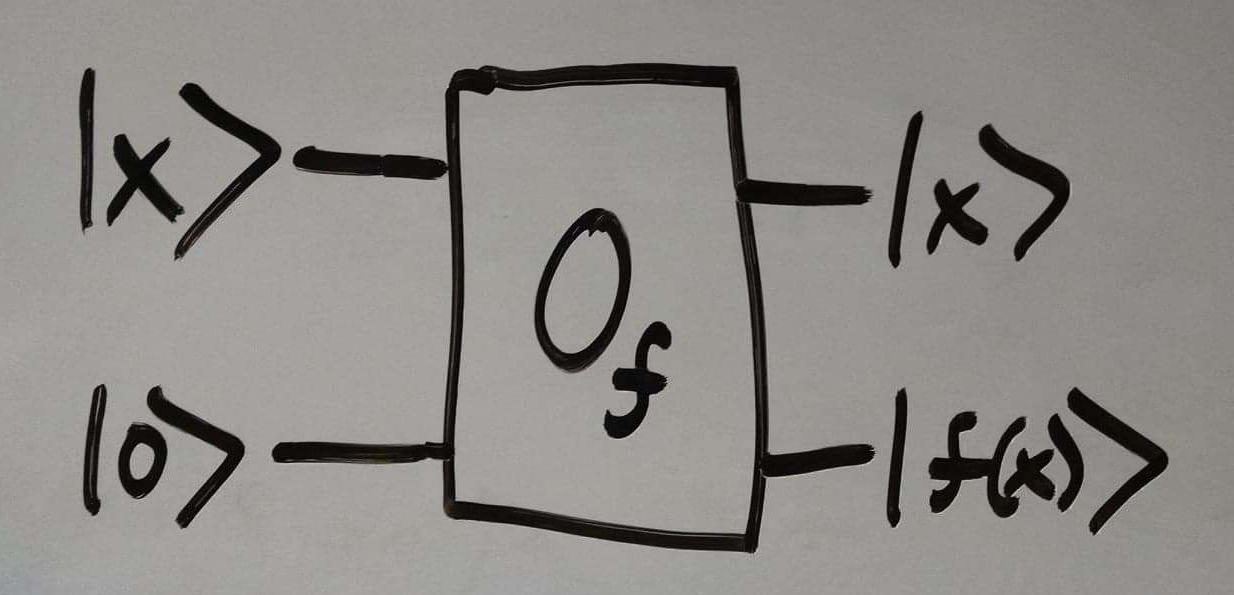In other words, we start with N qubits encoding the input to x, as well as a “blank” qubit that starts as |0⟩. Then we leave the first N qubits unchanged, and encode the value of f(x) in the initially blank qubit.

## 3. Flipping signs

Finally, we’ll use a clever trick. Let’s take a second look at the ⊕ gate.Then we get:Let’s consider both cases, f(x) = 0 and f(x) = 1.Also we can notice that we can get the state |y⟩ by applying a Hadamard gate to a qubit in the state |1⟩. Thus we can draw:## Putting it all together

We combine everything we learned so far in the following way:If we now ignore the last two qubits, as they were only really of interest to us for the purposes of building U, we get:And there we have it! We have built the quantum gate Uf that we used in the last two posts.# Grover’s algorithm

This is part 4 of my series on quantum computing. The earlier posts provide necessary background material.
Part 1: Quantum Computing in a Nutshell
Part 2: More on quantum gates
Part 3: Deutch-Josza Algorithm

# Grover’s algorithm

This algorithm involves searching through an unsorted list for a particular item. Let’s first look at how this can be optimally solved classically.

1. apple
2. banana
3. grapefruit
4. kiwi
5. guava
6. mango
7. lemon
8. papaya

A friend of yours wants to know where on the list they could find guava. They are allowed to ask you questions like “Is the first item on the list guava?” and “Is the nth item on the list gauva?”, for any n they choose. Importantly, they have no information about the ordering of your list.

How many queries do they need to perform in order to answer this question?

Well, since they have no information about its placement, they can do no better than querying random items in the list and checking them off one by one. In the best case, they find it on their first query. And in the worst case, they find it on their last query. Thus, if the list is of length N, the number of queries in the average case is N/2.

Grover’s algorithm solves the same problem with roughly √N queries. This is only a quadratic speedup, but still should seem totally impossible. What this means is that in a list of 1,000,000 items, you can find any item of your choice with only about 1,000 queries.

Let’s see how.

Firstly, we can think about the search problem as a function-analysis problem. We’re not really interested in what the items in the list are, just whether or not the item is guava. So we can transform our list of items into a simple binary function: 0 if the input is not the index of the item ‘guava’ and 1 if the input is the index of the item ‘guava’. Now our list looks like: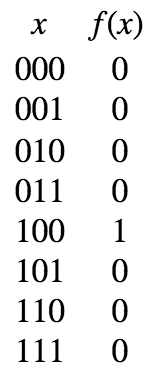Our challenge is now to find out for which value of x f(x) returns 1.

Now, this algorithm uses three quantum gates. Two of them we’ve already seen: HN and Uf. I’ll remind you what these two do:The third is called the Grover diffusion operator, D. In words, what D does to a state Ψ is reflect it over the average amplitude in Ψ. Visually, this looks like:Mathematically, this transformation can be defined as follows:Check for yourself that 2ā – ax flips the amplitude ax over ā. We are guaranteed that D is a valid quantum gate because it keeps the state normalized (the average amplitude remains the same after the flip).

Now, with HNU, and D in hand, we are ready to present the algorithm:We start with all N qubits in the state |00…0⟩, and apply the Hadamard gate to put them in a uniform superposition. Then we apply U to flip the amplitude of the desired input, and D to flip the whole superposition over the average. We repeat this step square root of the length of the list times, and then measure the qubits.

Here’s a visual intuition for why this works. Let’s suppose that N=3 (so our list contains 8 items), and the item we’re looking for is the 5th in the list (100 in binary). Here is each step in the algorithm:You can see that what the combination of U and D does to the state is that it magnifies the amplitude of the desired value of f. As you do this again and again, the amplitude is repeatedly magnified, reaching a maximum after sqrt(2N) repeats.

And that’s Grover’s algorithm! Once again we see that the key to it is the unusual phenomenon of superposition. By putting the state into superposition in our first step, we manage to make each individual query give us information about all possible inputs. And through a little cleverness, we are able to massage the amplitudes of our qubits in order that our desired output becomes overwhelmingly likely.

A difference between Grover’s algorithm and the Deutsch-Josza algorithm is that Grover’s algorithm is non-deterministic. It isn’t guaranteed with 100% probability of giving the right answer. But each run of the algorithm only delivers an incorrect answer with probability 1/2N, which is an exponentially small error. Repeating the algorithm n times gives you the wrong answer with only (1/2N)n probability. And so on.

While it is a more technically complicated algorithm than the Deutsch-Josza algorithm, I find Grover’s algorithm easier to intuitively understand. Let me know in the comments if anything remains unclear!

# Deutch-Josza Algorithm

Part 3 of the series on quantum computing.
Part 1: Quantum Computing in a Nutshell
Part 2: More on quantum gates

Okay, now that we’re familiar with qubits and some basic quantum gates, we’re ready to jump into quantum algorithms. First will be the Deutch-Josza algorithm, famous for its exponential speedup.

The problem to solve: You are handed a function f from {0,1}N to {0,1}, and guaranteed that it is either balanced or constant. A balanced function outputs an equal number of 0s and 1s across all inputs, and a constant function outputs all 0s or all 1s. For instance, if N = 3:f1 is balanced, and f2 is constant.

You are given an oracle that allows you to query f on an input of your choice. Your goal is to find out if f is balanced or constant with as few queries to the oracle as possible.

Let’s first figure out the number of queries classically required to solve this problem.

If f is balanced, then the minimum amount of queries required to determine that it is not constant is two – a 0 and a 1. And the worst case is that f is constant. In this case, we can only be sure it is not balanced by searching through half of all possible inputs, plus one. (For instance, we might get the series of outputs 0, 0, 0, 0, 0.)

So, since the number of possible inputs is 2N, the number of queries is between 2 and 2N-1 + 1. The average case thus involves roughly 2N-2 queries.

How about if we try to go about solving this problem with a quantum computers? How many queries to the oracle need we perform in order to determine if the function is balanced or constant?

Just one!

It turns out that there exists an algorithm that uses the oracle a single time, and always determines with 100% confidence whether f is balanced or constant.

Let’s prove it.

The algorithm uses only two different gates: the Hadamard gate H, and the quantum version of the oracle gate.

We’ll call the oracle gate Uf. Acting on the qubit state |x⟩, it returns (-1)f(x)|x⟩. That is, it flips the sign of the state if and only if evaluating f on the input x outputs 1. Otherwise it leaves the state of the qubits unchanged. So we have: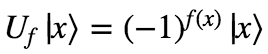In addition, we will use the general N-qubit Hadamard gate HN. We discussed how to get the matrix for the 2-qubit Hadamard gate last time. The general form of the Hadamard gate is:(You can prove this by showing it’s true for N=1, and then showing that if it’s true for N, then it’s true for N+1.)

Without further ado, here is the Deutch-Josza algorithm:In words, you start with all N qubits in the state |00…0⟩. Then you apply HN to all of them. Then you apply Uf, and finally HN again.

Upon measurement, if you find the qubits in the state |00…0⟩, then f is a constant function. If you find them in any other state, then f is guaranteed to be a balanced function.

Why is this so? Let’s prove it by tracking the states through each step.

At first, the state is |00…0⟩. After applying the Hadamard gate, we get: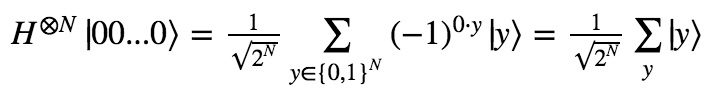In words, the qubit enters a uniform superposition over all possible states.

Now, applying Uf to the new state, we get:And finally, applying the Hadamard gate to this state, we get: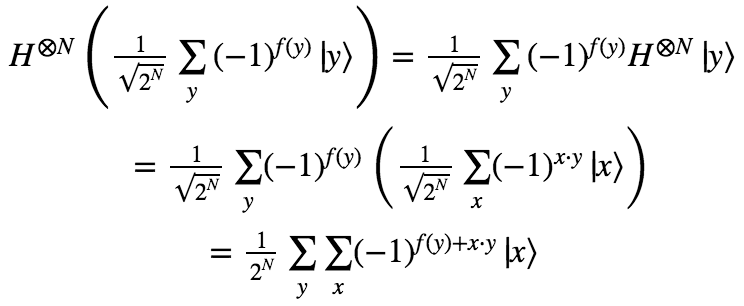For the purposes of this algorithm, we’re really only interested in the amplitude of the |00…0⟩ state. This amplitude is simply:What we want to show is that the square of this amplitude is 0 if f is balanced and 1 if f is constant. (I.e. the probability of observing |00…0⟩ is 0% for a balanced function and 100% for a constant function). Let’s look at both cases in turn.

### Case 1: f is constant

If f is constant, than all the terms in the sum are the same: either 1/2N or -1/2N. Since there are 2N values for y to be summed over, the amplitude is either +1 or -1. And since the probability is the amplitude squared, this probability is guaranteed to be 100%!

### Case 2: f is balanced

If f is balanced, then there are an equal number of 1/2N terms and -1/2N terms. I.e. every 1/2N term cancels out a -1/2N term. Thus, the amplitude is zero, and so is the probability!

In conclusion, we’ve now seen how a quantum computer can take a problem that can only be classically solved with an average of 2N-2 queries, and solves it with exactly 1 query. I.e. quantum algorithms can provide exponential speedups over classical algorithms!

Looking carefully at the inner workings of this algorithm, you can get a clue as to how it achieves this speedup. The key is in the ability to superpose a single qubit into multiple observable states. This allowed us to apply our oracle operator to all possible inputs at once, in exactly one step.

Somebody that believed in the many-worlds interpretation of quantum mechanics would say that when we put our qubit into superposition, we were creating 2N worlds, each of which contained a different possible input value. Then we leveraged this exponential number of worlds for our computational benefit, applying the operation in all the worlds at once before collapsing the superposition back to one single world with the final Hadamard gate.

It’s worth making a historical note here. David Deutsch was one of the two people that discovered this algorithm, which was the first example where a quantum computer would be able to surpass the ordinary limits on classical computers. He is also a hardcore proponent of the many-worlds interpretation, and has stated that the fact that quantum computers can do more than classical computers is strong evidence for this interpretation. His argument is that the only possible explanation for quantum computers surpassing the classical limits is that some of the computation is being offloaded into other universes.

Anyway, next we’ll look at a more famous algorithm, Grover’s algorithm. This one only provides a quadratic (not exponential) speed up. However, it solves a more useful problem: how to search through an unsorted list to find a particular item. See you next time!

Next: Grover’s algorithm

# More on quantum gates

In the last post, we described what qubits are and how quantum computing involves the manipulation of these qubits to perform useful calculations.

In this post, we’ll abstract away from the details of the physics of qubits and just call the two observable states |0⟩ and |1⟩, rather than |ON⟩ and |OFF⟩. This will be useful for ultimately describing quantum algorithms. But before we get there, we need to take a few more steps into the details of quantum gates.

Recap: the general description of a qubit is |Ψ⟩ = α|0⟩ + β|1⟩, where α and β are called amplitudes, and |α|² and |β|² are the probabilities of observing the system in the state |0⟩ and |1⟩, respectively.

We can also express the states of qubits as vectors, like so:Quantum gates are transformations from quantum states to other quantum states. We can express these transformations as matrices, which when applied to state vectors yield new state vectors. Here’s a simple example of a quantum gate called the X gate:Applied to the states |0⟩ and |1⟩, this gate yieldsApplied to any general state, this gate yields:Another gate that is used all the time is the Hadamard gate, or H gate.Let’s see what it does to the |0⟩ and |1⟩ states:In words, H puts ordinary states into superposition. Superposition is the key to quantum computing. Without it, all we have is a fancier way of talking about classical computing. So it should make sense that H is a very useful gate.

One more note on H: When you apply it to a state twice, you get back the state you started with. A simple proof of this comes by just multiplying the H matrix by itself:Okay, enough with single qubits. While they’re pretty cool as far as they go, any non-trivial quantum algorithm is going to involve multiple qubits.

It turns out that everything we’ve said so far generalizes quite nicely. If we have two qubits, we describe the combined system by smushing them together with what’s called a tensor product (denoted ⊗). What this ends up looking like is the following:The first number refers to the state of the first qubit, and the second refers to the state of the second.

Let’s smush together two arbitrary qubits: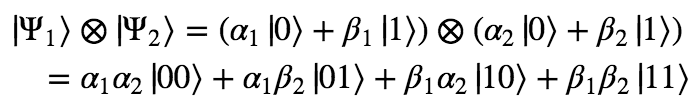This is pretty much exactly what we should have expected combining qubit states would look like.

The amplitude for the combined state to be |00⟩ is just the product of the amplitude for the first qubit to be |0⟩ and the second to be |0⟩. The amplitude for the combined state to be |01⟩ is just the product of the amplitude for the first qubit to be |0⟩ and second to be |1⟩. And so on.

We can write a general two qubit state as a vector with four components.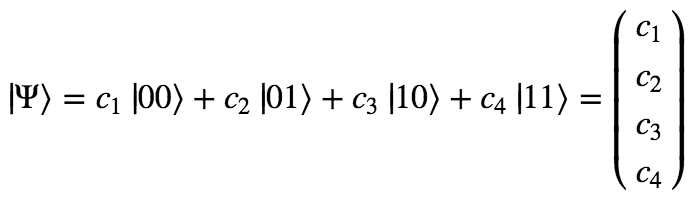And as you might expect by now, two-qubit gates are simply 4 by 4 matrices that act on such vectors to produce new vectors. For instance, we can calculate the 4×4 matrix corresponding to the action of a Hadamard gate on both qubits:Why the two-qubit Hadamard gate has this exact form is a little beyond the scope of this post. Suffice it to say that this is the 4×4 matrix that successfully transforms two qubits as if they had each been put through a single-qubit Hadamard gate. (You can verify this for yourself by simply applying H to each qubit individually and then smushing them together in the way we described above.)

Here’s what the two-qubit Hadamard gate does to the four basic two-qubit states.Here’s a visual representation of this transformation using bar graphs: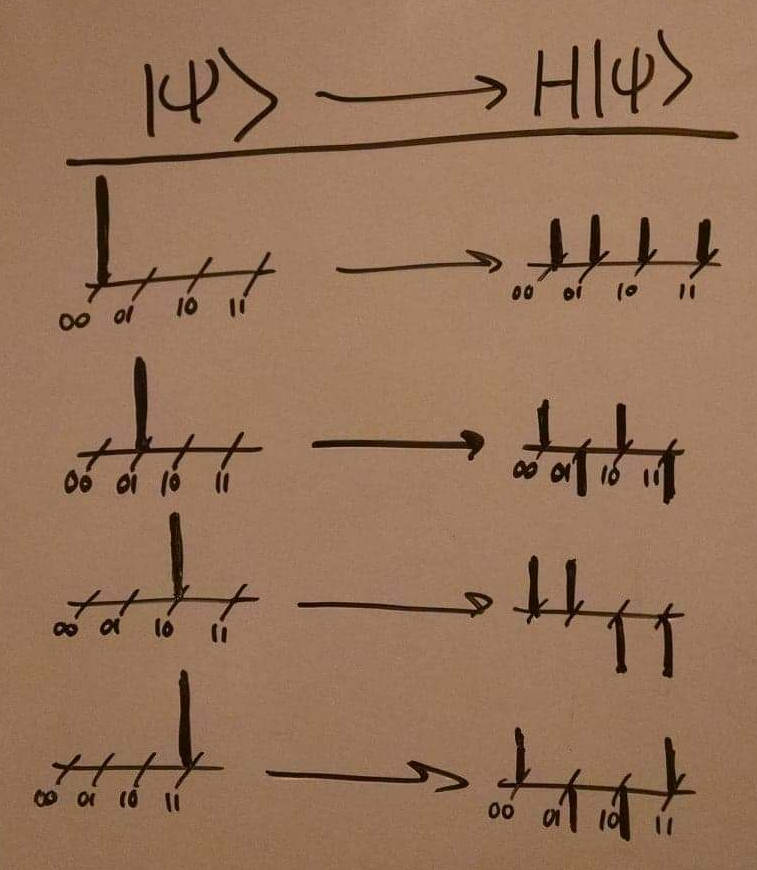We can easily extend this further to three, four, or more qubits. The state vector describing a N-qubit system must consider the amplitude for all possible combinations of 0s and 1s for each qubit. There are 2ᴺ such combinations (starting at 00…0 and ending at 11…1). So the vector describing an N-qubit system is composed of 2ᴺ complex numbers.

If you’ve followed everything so far, then we are now ready to move on to some actual quantum algorithms! In the next post, we’ll see first how qubits can be used to solve problems that classical bits cannot, and then why quantum computers have this enhanced problem-solving ability.

# Quantum Computing in a Nutshell

You can think about classical bits as like little switches. They have two possible states, ON and OFF, and are in either one or the other.Then we have logic gates, which are like operations we perform on the switches. If we have one switch, we might flip it from whatever state it’s in to the opposite state. If we have two, we might flip the second switch conditional on the first switch being ON, and otherwise do nothing. And so on.Put together a bunch of these bits and gates and you get an algorithm. If you arrange them cleverly enough, you end up getting interesting computations like adding binary numbers and factoring primes and playing Skyrim.

Quantum computing is a fundamentally different beast. To start with, the fundamental units of quantum computation are non-deterministic. We call them qubits, for quantum bits. While a qubit can, like a classical bit, be in the state ON and the state OFF, it can also be in other more exotic states:Important! Just like a classical bit, a qubit will only ever be observed in two possible states, ON and OFF. We never observe a qubit being in the state “36% ON and 64% OFF.” But if we prepare a qubit in that particular state, and then measure it over and over again, we could find that 36% of the time it is ON and 64% of the time it is OFF. That is, the state of a qubit specifies the probability distribution over possible observed outcomes.

Let’s compare the complexity of bits to the complexity of qubits.

To specify the state of a classical bit, we only need to answer a single yes or no question: Is it ON?

To specify the state of a quantum bit, we need to answer a more complicated question: What is the probability that it is ON?

Since probabilities are real numbers, answering the second question requires infinitely more information than answering the first. (In general, specifying a real number to perfect precision requires answering an unbounded number of yes or no questions.) The implication of this is that in some sense, the state of a qubit contains infinitely more information than the state of a classical bit. The trick of quantum computing is to exploit this enhanced information capacity in order to build quantum algorithms that beat out the best classical algorithms.

Moving on! The set of all states that a qubit can be in is the set of all probability distributions over the outcomes ON and OFF.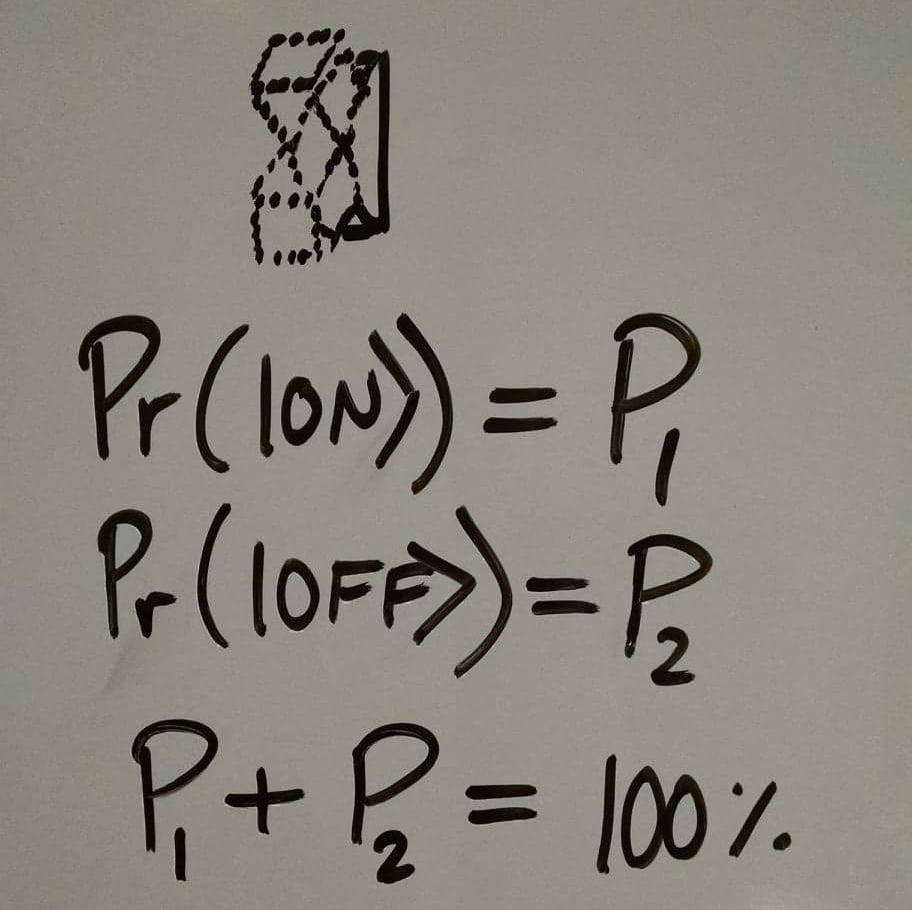Now, we could describe a simple probabilistic extension to classical computation, where logic gates transform probability distributions into different probability distributions, and algorithms neatly combine these gates to get useful computations, and be done with it. But it turns out that things are a slight bit more complicated than this. Having described this probabilistic extension, we would still not have quantum computing.

In fact, the states of qubits are specified not by probability distributions over the observations ON and OFF, but an amplitude distribution over these observations.

What are these amplitudes? An amplitude is simply a thing that you square to get the probability of the observation. Amplitudes have a wider range of possible values than probabilities. While a probability has to be greater than zero, an amplitude can be negative (since the square of a negative number will still be positive). In fact, amplitudes can even be complex numbers, in which case the square of the amplitude is just the square of its distance from zero in the complex plane.Now, why does it matter that the set of quantum states be described by amplitudes rather than probabilities? After all, the amplitudes will always just be converted back to probabilities when we observe the qubits, so what difference does it make if the probability came from a negative amplitude or a positive one? The answer to this question is difficult, but it comes down to this:

For some reason, it appears that on the quantum level, the universe calculates the states of particles in terms of these complex numbers we call amplitudes, not probabilities. This was the discovery of experimentalists in the 1900s that looked at things like the double slit experiment, the Stern-Gerlach experiment, and so on. If you try to just analyze everything in terms of probabilities instead of amplitudes, you will get the wrong answer.

We’ll write the state of a qubit that has an amplitude alpha of being ON and an amplitude beta of being OFF as follows:It’s useful to have a few different ways of visualizing the state of a qubit.Now, quantum gates are transformations that take amplitude distributions to different amplitude distributions.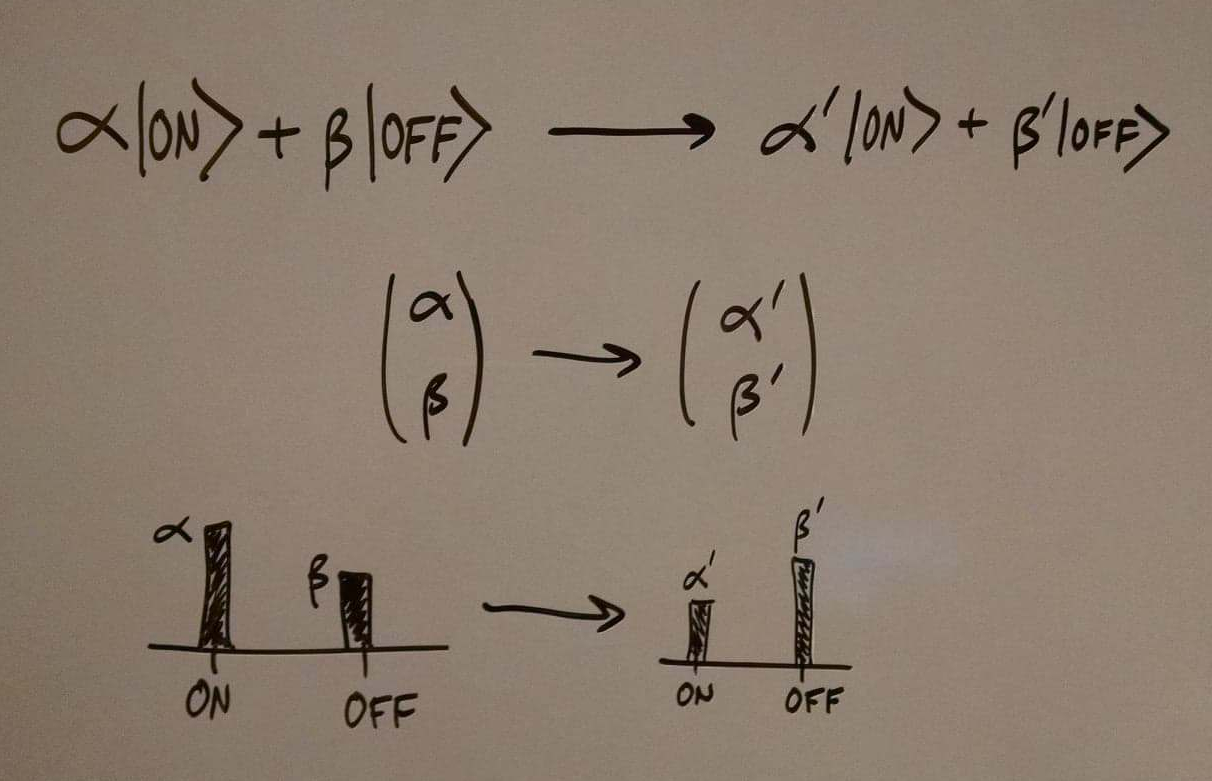The set of physically realizable quantum gates is the set of all transformations that take normalized states (|α|² + |β|² = 1) to other normalized states. Some of these gates are given special names like the Hadamard gate or the CNOT gate and crop up all the time. And just like with classical states, you can put together these gates in clever ways to construct algorithms that do interesting computations for you.

So, that’s quantum computing!

Now, the first thing to notice is that the set of all things that quantum computers can do must contain the set of all things that classical computers can do. Why? Because classical computers are just a special case of quantum computers where states are only allowed to be either ON or OFF. Every classical logic gate has a parallel quantum logic gate, and so every classical algorithm is a quantum algorithm is disguise.

But quantum computers can also do more than classical computers. In the next posts, I’ll give two examples of quantum algorithms we’ve discovered that solve problems at speeds that are classically impossible: the Deutsch-Jozsa algorithm and Grover’s algorithm. Stay tuned!

(Next: More on quantum gates)

# Matter and interactions in quantum mechanics

Here’s another post on how quantum mechanics is weird.

Let’s consider the operation of swapping around different quantum particles. If the coordinates of the first particle are denoted x1 and the second denoted x2, we’ll be interested in the transformation:

Ψ(x1, x2) → Ψ(x2, x1)

Let’s just give this transformation a name. We’ll call it S, for “swap”. S is an operator that is defined as follows:

S Ψ(x1, x2) = Ψ(x2, x1)

Now, clearly if you swap the particles’ coordinates two times, you get back the same thing you started with. That is:

S2 Ψ(x1, x2) = Ψ(x1, x2)

This implies that S2 is an operator with eigenvalue +1. What does this tell us about the spectrum of eigenvalues of S?

Suppose S has an eigenvalue λ. Then, from the above, we see:

S2 Ψ = Ψ
S SΨ = Ψ
S λΨ = Ψ
λ SΨ = Ψ
λ2 Ψ = Ψ

So λ = ±1.

In other words, the only possible eigenvalues of S are +1 and -1.

This tells us that the wave functions of particles must obey one of the following two equations:

SΨ = +Ψ
or
SΨ = -Ψ

Said another way…

Ψ(x1, x2) = Ψ(x2, x1)
or
Ψ(x1, x2) = -Ψ(x2, x1)

This is pretty important! It says that by the nature of what it means to swap particles around and the structure of quantum mechanics, it must be the case that the wave function obtained by switching around coordinates is either the same as the original wave function, or its negative.

This is a huge constraint on the space of functions we’re considering; most functions don’t have this type of symmetry (e.g. x2 + y ≠ y2 + x).

So we have two possible types of wave functions: those that flip signs upon coordinate swaps, and those that don’t. It turns out that these two types of wave functions describe fundamentally different kinds of particles. The first type of wave functions describes fermions, and the second type describes bosons.

All particles in the standard model of particle physics are either fermions or bosons (as expected by the above argument, since there are only two possible eigenvalues of S). Fermions include electrons, quarks, and neutrinos. Bosons include photons, gravitons, and the Higgs boson. This sampling of particles from each category should give you the intuitive sense that fermions are “matter-like” and bosons “force-like.”

Of course, the definition of fermions and bosons we gave above doesn’t reference matter-like or force-like behavior. Somehow the qualitative difference between fermions and bosons must arise from this simple sign difference. How?

We’ll start exploring this by examining the concept of non-separability of wave functions.

A wave function is separable if it is possible to write it as a product of two individual wave functions, one for each coordinate:

Ψ(x1, x2) = Ψ1(x1) Ψ2(x2)

This is a really nice property. For one thing, it allows us to solve the Schrodinger equation extremely easily by using the differential equations method of variable separation. For another, it tells us that the wave function is simple in a way. If we’re interested in only one set of coordinates, say x1, then we can easily disregard the whole rest of the wave function and just look at Ψ1.

But what does it mean? Well, if a wave function is separable, then it is possible to sensibly ask questions about the properties of individual particles, independent of each other. Why? Because you can just look at the component of the wave function corresponding to the particle you’re interested in, and the rest behaves just like a constant for all you care.

If a wave function is non-separable (i.e. if there aren’t any two functions Ψ1 and Ψ2 for which Ψ(x1, x2) = Ψ1(x1) Ψ2(x2)), then the story is trickier. For all intents and purposes, it loses meaning to talk about one particle independent of the other.

This is hard to wrap our classical brains around. If the wave function cannot be separated, then the particles just don’t have positions, momentums, and so on that can be described independently.

Now, it turns out that most of matter is composed of non-separable wave functions. Pretty much any time you have particles interacting, their wave function cannot be written as a product of independent particles. A lot of the time, wave functions are very approximately separable (consider the wave function describing two electrons light years away). But in such cases, when we talk about the two electrons as two distinct entities, we’re really using an approximation that is not fundamentally correct.

Now, this all relates back to the fermion/boson distinction in the following way. Suppose we had a system that was described by a separable wave function.

Ψ(x1, x2) = Ψ1(x1) Ψ2(x2)

Now what happens when we apply the swap operator to Ψ?

S Ψ(x1, x2) = Ψ(x2, x1) = Ψ1(x2) Ψ2(x1)

As we’d expect, we get the particle described by x2 in the wave function initially occupied by the particle described by x1, and vice versa. But now, our system must obey one of two constraints:

Since SΨ = ±Ψ,

Ψ1(x2) Ψ2(x1) = Ψ1(x1) Ψ2(x2)
or
Ψ1(x2) Ψ2(x1) = – Ψ1(x1) Ψ2(x2)

Let’s take the second possibility for fermions first. It turns out that this constraint can never be satisfied. Why? Look:

Suppose f(x) g(y) = – f(y) g(x)
Then we have:  f(x)/g(x) = -f(y)/g(y)

Since the left is a pure function of x, and the right of y, this implies that they are both equal to a constant.
I.e. f(x)/g(x) = k, for some constant k.
But then f(y)/g(y) = k as well.

Thus k = -k,
which can only be true if k = 0.

This argument tells us that the only function that satisfies (1) Ψ describes a fermion and (2) Ψ is separable, is Ψ(x1, x2) = 0. Of course, this is not a wave function, since it is not normalizable. In other words, fermion wave functions are always non-separable!

What about boson wave functions? The equation

Ψ1(x2) Ψ2(x1) = Ψ1(x1) Ψ2(x2)

does have some solutions, but they are highly constrained. Essentially, for this to be true for all x1 and x2, Ψ1 and Ψ2 must be the same function.

In other words, bosons are separable only in the case that their independent wave functions are completely identical.

So. If fermions cannot be described by a separable wave function, how can we describe them? We can be clever and notice the following:

While Ψ(x1, x2) = Ψ1(x1) Ψ2(x2) does not obey the requirement that SΨ = -Ψ,
Ψ(x1, x2) = Ψ1(x1) Ψ2(x2) – Ψ2(x1) Ψ1(x2) does!

Let’s check and see that this is right:

S Ψ(x1, x2)
= S [Ψ1(x1) Ψ2(x2) – Ψ2(x1) Ψ1(x2)]
= Ψ1(x2) Ψ2(x1) – Ψ2(x2) Ψ1(x1)
= -Ψ(x1, x2).

Aha! It works.

Now we have a general description for fermion wave functions that does not violate the swap constraints. We can do something similar for bosons, giving us:

Fermions
Ψ(x1, x2) = Ψ1(x1) Ψ2(x2) – Ψ2(x1) Ψ1(x2)

Bosons
Ψ(x1, x2) = Ψ1(x1) Ψ2(x2) + Ψ2(x1) Ψ1(x2)

(Note: These are not all possible fermion/boson wave functions. They only capture one set of allowable wave functions.)

Now, let’s notice a peculiar feature of the fermion wave function. What happens if we ask for the probability amplitude of the two particles being at the same place at the same time? We find out by taking the fermion equation and setting x1 = x2 = x:

Ψ(x, x) = Ψ1(x) Ψ2(x) – Ψ2(x) Ψ1(x)
= 0

More generally, we can prove that any fermion wave function must have this same property.

SΨ(x1, x2) = -Ψ(x1, x2)
Ψ(x2, x1) = -Ψ(x1, x2)
Ψ(x, x) = -Ψ(x, x)
Ψ(x, x) = 0

Crazy! Apparently, two fermions cannot be at the same position. And since wave functions are smooth, fermions will generally have a small probability of being close together.

This is the case even if the fermions are interacting by a strong attractive force. It’s almost as if there is an intrinsic repulsive force keeping fermions away from each other. But this would be the wrong way to think about it. This feature of the natural world is not discovered by exploring different possible forces and potentials. Instead we got here by reasoning purely abstractly about the nature of swapping particles. We are a priori guaranteed this unusual feature: that if fermions exist, they must have zero probability of being located at the same point in space.

The name for this property is the Pauli exclusion principle. It’s the reason why electrons in atoms spread out in ever-larger orbitals around nuclei instead of all settling down to the nucleus. It is responsible for the entire structure of the periodic table, and without it we couldn’t have chemistry and biology.

(Quick side note: You might have thought of something that seems to throw a wrench in this – namely, that the ground state of atoms can hold two electrons. In general, electron orbitals in atoms are populated by more than one electron. This is possible because the wave function has extra degrees of freedom such as spin, the details of which determine how many electrons can fit in the same spatial orbital. I.e. two fermions can have the same spatial amplitude distribution, so far as they have some other distinct property.)

Mathematically, this arises because of interference effects. As the two fermions get closer and closer to each other, their wave functions interfere with one another more and more, turning their joint probability to zero. Fermions destructively interfere.

And bosons? They constructively interfere! At x1 = x2 = x, for bosons, we have:

Ψ(x, x) = Ψ1(x) Ψ2(x) + Ψ2(x) Ψ1(x)
= 2 Ψ1(x) Ψ2(x)

In other words, while fermions disperse, bosons cluster! Just like how fermions seemed to be pulled away from each other with an imaginary force, bosons seem to be pulled together! (Once again, this is only a poetic description, not to be taken literally. There is no extra force concentrating bosons, as can be evidenced by the straight trajectory of parallel beams of light).

I’m not aware of a name for this principle to complement the Pauli exclusion principle. But it explains phenomena like lasers, where enormous numbers of photons concentrate together to form a powerful beam of light. By contrast, an “electron laser” that concentrates enormous numbers of electrons into a single beam would be enormously difficult to create.

The intrinsic tendency for fermions to repel each other leads them to form complicated structures that spread out through space, and give them the qualitative material resistance to objects passing through them. You just can’t pack them arbitrarily close together – eventually you’ll run out of degrees of freedom and the particles will push each other away.

Bosons, on the other hand, are like ghosts – they can vanish into each others wave functions, congregate together in large numbers and behave as a single entity, and so on.

These differences between fermions and bosons pop straight out of their definitions, and lead us to the qualitative differences between matter and forces!